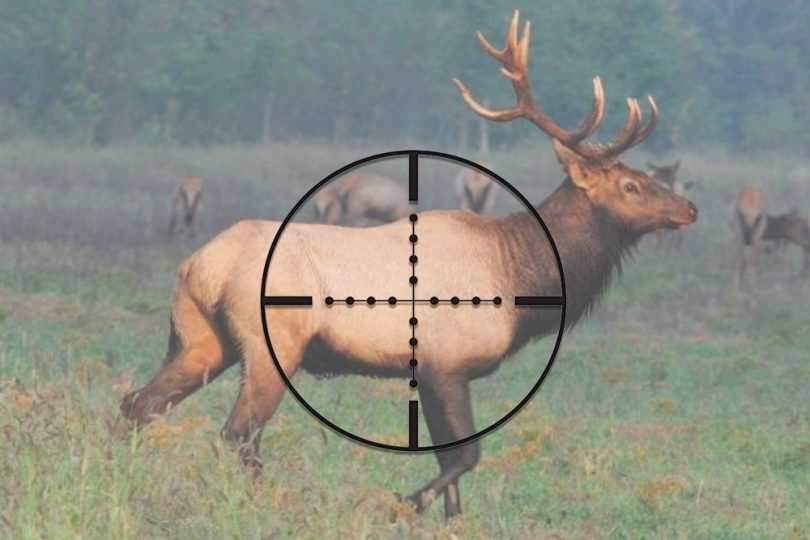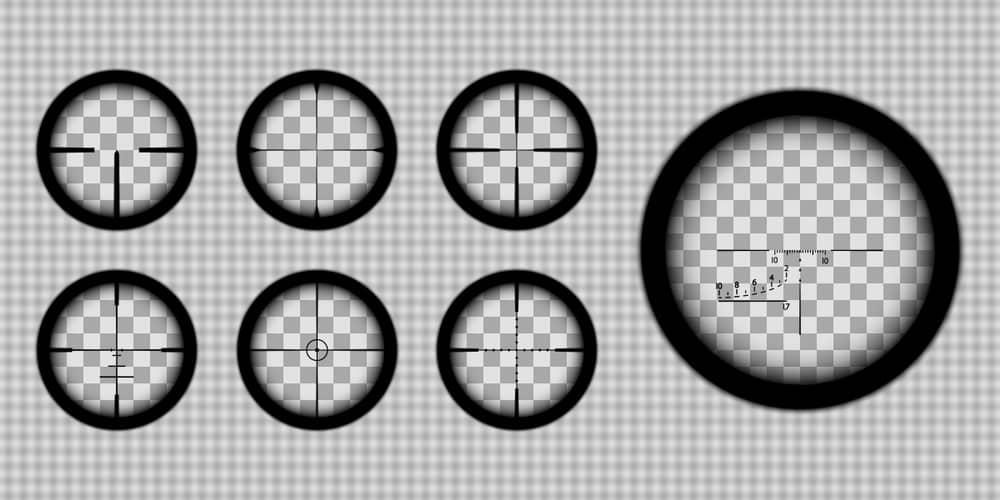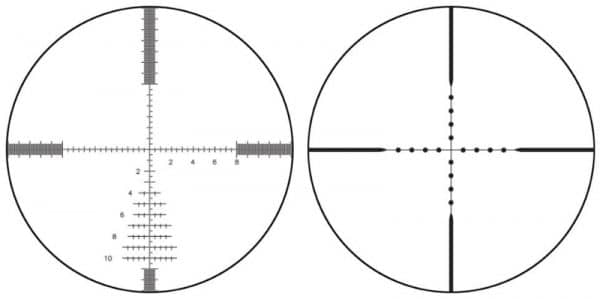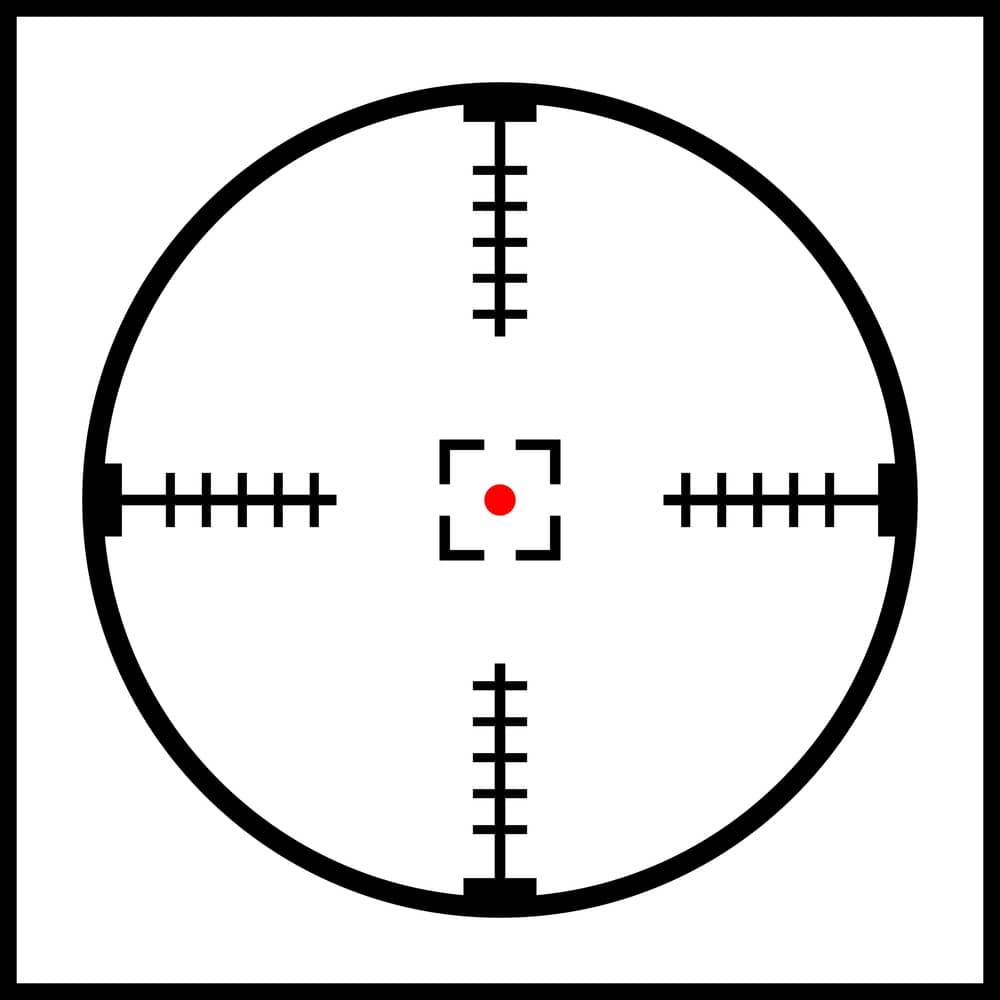Rangerexpert is audience-supported. When you buy through links on this site, we may earn an affiliate commission that we use for site maintenance. Learn more

Blogs

# How to Use Mil Dot Scopes? – Everything You Need to KnowWritten by
Last Update: August 11, 2023

Mil Dot scopes are preferred by renowned military forces (including NATO) for their precision and simplicity.

Many riflescopes have a rangefinding reticle of one type or another. The most common one is the Mil-dot Reticle.

This guide will teach you how to use mil dot scopes correctly. We’ll also explore the basics of mill measurement and how to zero a mil dot scope to ensure accuracy and precision every time you take a shot.

Let’s explore how to use a mil dot scope for dummies so even a beginner can shoot like a Pro!## Basics of Rifle Scopes

Rifle scopes are telescopic aiming devices used to spectate the target from a distance. They are known for producing a precise and vivid image of the target from various distances.

Some primary scope components are lenses (objective and ocular), turret (windage and elevation), reticle, power selector, erector lens, scope tube, and eyepiece.

The larger objective lens collects the light around the target and transmits it to the smaller ocular lens through the erector lens. The ocular lens offers a clear horizon to the user.

Windage and elevation turrets are two knobs for reticle adjustments. Windage is responsible for horizontal, while elevation is for the vertical direction.

The power selector controls the magnification setting (zoom in or out) range.

The reticle is another name for crosshair: two lines cross each other in the center to give the target’s focal point.

Different gun scopes have several types of reticles to focus on the target: fine, duplex, German, target, Mil Dot, bullet drop compensator, and many more.

The first and second focal planes are the two places to install the reticle.

FFP at the front of the power selector means the reticle will change during magnification. In contrast, SFP at the rear of magnification won’t change the reticle size.

## What is So Special about Mil-Dot Scopes?

Mil-Dot is one of the many reticles used in both short-and long-range scopes. It’s one of a kind because of its precision.

It offers calculations like the estimated range of the target, ballistic effect, hitting point as the size of the target, and more. Mil-Dot uses a manual optical device.

Its quick aiming and estimated distance are the most remarkable blends of the reticle. This reticle will assist you in crosswind shooting and when you lock a moving target.

Mil is a term used for milliradians, sometimes referred to as MRAD.

This unit of angular measurement equals a 1000th of a radian, so each number of mills represents 0.001 radians.

With this calculation, one yard equals 1000 yards, and one meter equals 1000 meters.

## The Reason You Need Mil dot Scopes

Mil dots are incredibly useful if you need to estimate the size or distance of a target, just like a hunting rangefinder.

However, you’ll need to know the distance beforehand, to calculate the size of the approaching target and vice versa.

Not only that, but it can also help you measure and adjust for wind drift. Once you know the direction and speed of the wind, it’s easy to calculate wind drift.

If you have all the ballistic information of your rifle and the bullet, mil-dots can also help you measure the accurate bullet drop over a known distance.

## How Does a Mil Dot Scope Work?When you are ready to target a specific range, you must go through all the calculations to achieve that perfect shot. Keep in view the ballistic impact and wind speed for the ideal shot.

Remember, mil captures the angular measurement, not the linear measurement.

It’s 1/ 6400th of a circle as per standard evaluation. So, a milliradian equals 1 yard at 1000 yards and 1 meter at 1000 meters.

Here’s the formula to find the range in a Mil Dot reticle scope:

(The width or height of the target in yards × 1000) / target’s width or height in mils = range (in yards)

The meter formula is the same, but all measurements should be in meters, not yards.

(The width or height of the target in meters × 1000) / target’s width or height in mils = range (in meters)

For example, if a target object is 2 yards in height, and covers 1.5 lengths in mils, then the range equals

(2×1000)/ 1.5 = 1333.34 yards. This is how to use mill dot scopes most easily and efficiently.

## How to Measure Distance To Your Target Accurately?

For this, you need to understand the pattern of the reticle.

There are several dots on it; they are at 1 mil distance when measured from half of one dot to half of another.

1 mil dot equals 3.6” or 10 cm at a 100 yards distance.

So, you need to make a 1 mil adjustment on the scope and learn about the horizontal direction at a known difference.

 Distance (yards) 100 200 300 400 500 1 mil size (inches) 3.6 7.2 10.8 14.4 18 Distance (meters) 100 200 300 400 500 1 mil size (centimeters) 10 20 30 40 50

And so on.

The formula for 1 mil size adjustment at any defined distance (in inches):

(Distance (yards) × 3.6 (inches))/100 = inches on which 1 mil size is adjusted

Example: (720×3.6)/100 = 25.92”

The formula for 1 mil size adjustment at any defined distances (in centimeters):

(Distance (meters))/10 = centimeters on which 1 mil size is adjusted

Example: (1450)/10 = 145 cm

## How to Use Mil Dot Scopes?Using Mil-Dots in a hunting scope is a challenge.

Nowadays, people lean towards a laser range finding tool (Laser Rangefinder) to estimate the distance. Still, a Mil-Dot can come to the rescue when the rangefinder fails.

The formula for estimated distance in yards:

(The target size (in inches) × 27.778 (a constant for calculating distance in yards)) / mil size = estimated distance (yards)

The formula for estimated distance in meters:

(The target size (in cm) × 10 (a constant for calculating distance in meters)) / mil size = estimated distance (meters)

For example, if an object on the reticle is 15″ or 38.1 cm tall and spreads to almost 1.5 mil space on the reticle, then use this formula to calculate the distance.

In yards:

(15” ×27.778) / 1.5 = 416.67 / 1.5 = 277.78 yards

In meters:

(38.1 cm ×10) / 1.5 = 381/ 1.5 = 254 meters

If you don’t know how to use mill dot scopes for hunting, use the formulas mentioned above to accurately measure the distance between you and your prey.

### Making Adjustments with a Mil Dot Scope

The most common adjustment is 0.1 or 1/10th per Mil Dot scope. This adjustment will offer you 10 clicks per Mil-Dot.

Formula: Bullet drop as per yards / 1 mil size as per yards = mil adjustment

 Distance (yards) 100 200 300 400 500 Bullet drop (inches) 0 4.2 15.7 37.6 73 1 mil size (inches) 3.6 7.2 10.8 14.4 18 Mil adjustment 0 0.6 1.5 2.6 4.1 Turret clicks 0 6 15 26 41

Example: At 300 yards, you’ll get 15.7 inches bullet drop.

15.7”/10.8”= 1.5 mil

So, the Mil-Dot difference is 1.5 inches; then, this adjustment will give 15 clicks.

You can go further with the formula for centimeters calculation: change that centimeters calculation into inches and apply the same formula.

Although, an online calculator is better.

### What About The Bullet Drop?

Bullet drop shows the exact dropping limit at a specific range or distance.

To precisely calculate your aiming point and hitting point at the drop rate, you need to know about your rifle and ballistic effect.

Numerous online calculators are available to get a precise number for bullet drops.

If you want to shoot within 100 yards, you won’t face any bullet drops. However, keep in mind that it’ll gradually increase every 100 yards.

 Distance (yards) 100 200 300 400 500 Bullet drop (inches) 0 4.2 15.7 37.6 73 Mil adjustment 0 0.6 1.5 2.6 4.1 Distance (meters) 100 200 300 400 500 Bullet drop (centimeters) 0 14 51.7 122.37 242.3 Mil adjustment 0 0.7 1.7 3.1 4.8

Bullet drop / fixed 1 mil size for the distance (as 3.6 per 100 yards) = required adjustment

Example: For 300 yards, the trajectory will be something like this:

15.7” / 10.8” = 1.5 is the required adjustment.

For centimeters calculation, you need to convert the centimeters into inches and apply the same formula.

## FAQs

### 1. What‘s better – Mil-Dot or MOA?

Ans. Mil-Dot (milliradian) and MOA (minute of angle) have their pros and cons. A Mil-Dot provides 1/10 clicks, which is considered less fine than the MOA’s ¼ clicks adjustment.

### 2. How many inches are a mil at 100 yards?

Ans. There are 3.6 inches in a mil at 100 yards, but these inches will change when the yards change.

For example, at 200 yards, they’ll change into 7.2 inches. In meters, 1 mil equals 10 cm at 100 yards.

### 3. What does the military use – Mil or MOA?

Ans. The military uses mil mostly, but definitely, they haven’t forgotten about their best blend, from the 70s and 80s, of the mil-based reticle and MOA knobs.

### 4. What does a Mil Dot reticle look like?

Ans. MIL Dot refers to a specific pattern of duplex crosshair reticles. The reticle has four 0.25 mil diameter dots placed on each axis. The arrangement of these dots allows you to estimate the range.

### 5. What is a more accurate MOA or MIL?

Ans. 1/10 MIL adjustments are less precise than 1/4 MOA but the MIL values are easier to communicate.

If you calculate in meters/cm, MIL will be easier for you. MOA will be the right choice if you prefer yards/inches for range estimation.

### 6. What is the difference between MIL Dot and MRAD?

Ans. The military MIL works by dividing the circle into even numbers. The number splits into decimal fractions. MRAD is the scientific MIL that represents a 1000th of a radian. This gives you an odd value for a full circle.